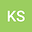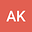Modified subgradient extragradient method for approximating of the system of general equilibrium problem and fixed point problem
••• Kanyanee Saechou,
• Atid KANGTUNYAKARN
Kanyanee Saechou
King Mongkut\'s Institute of Technology Ladkrabang
Author ProfileAtid KANGTUNYAKARN
King Mongkut\'s Institute of Technology Ladkrabang
Author Profile## Abstract

In this paper, we introduce the system of general equilibrium problem (SGEP) and new subgradient extragradient by using the concept of the set of solution of the modified variational inequality problem introduced by \cite{kangtunyakarn}. Then, we establish and prove weak and strong convergence theorem of the new subgradient extragradient algorithm for finding the set of the solutions of the SGEP under some suitable conditions of $\alpha_{n}$ and $\beta_{n}$ with $\alpha_{n}+\beta_{n}\leq 1$. Moreover, we apply our main theorem to prove weak and strong convergence theorems for finding solutions of the generalized equilibrium problem, the system of equilibrium problem, the variational inequality problem and the general system of variational inequality problem. In the last section, we give three numerical examples to support our main result.